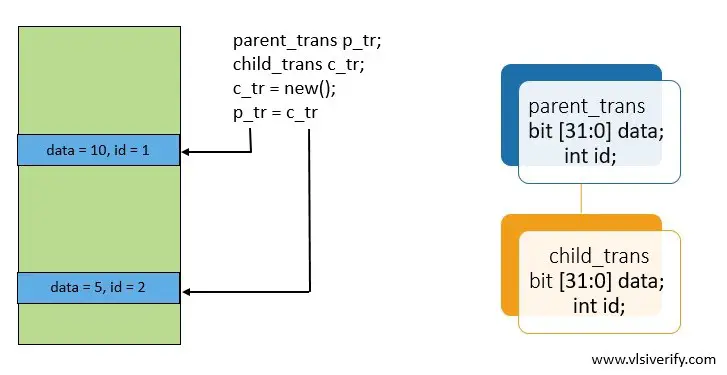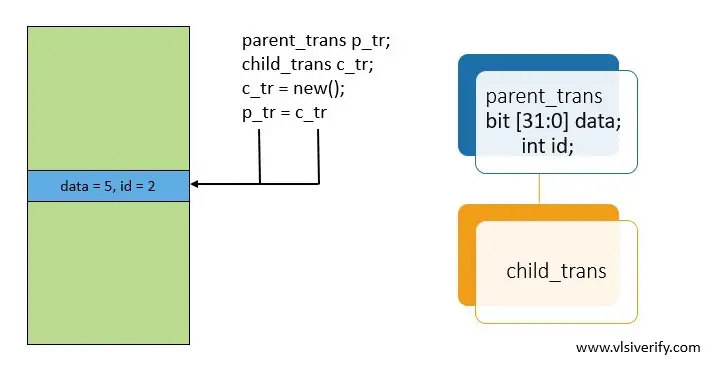Tutorials

Polymorphism means having many forms. A base class handle can invoke methods of its child class which has the same name. Hence, an object can take many forms.

1. As we know, the derived class object can override methods of its base class. Similarly, the base class object can also override the method of one of the child classes. It means a base class method has different forms based on derived class implementation.
2. To use many forms of the method, the virtual keyword must be used in the method definition.

## Polymorphism Example

In the below example, child_A, child_B, and child_C are derived from the parent class. All child class handles are assigned to the parent class handle. Using the polymorphism concept, the parent class handle can invoke child class methods as shown in example.

``````class parent;
bit [31:0] data;
int id;

virtual function void display();
\$display("Base: Value of data = %0d, id = %0d", data, id);
endfunction
endclass

class child_A extends parent;
function void display();
\$display("Child_A: Value of data = %0d, id = %0d", data, id);
endfunction
endclass

class child_B extends parent;
function void display();
\$display("Child_B: Value of data = %0d, id = %0d", data, id);
endfunction
endclass

class child_C extends parent;
function void display();
\$display("Child_C: Value of data = %0d, id = %0d", data, id);
endfunction
endclass

module class_example;
initial begin
parent p_A, p_B, p_C;
child_A c_A = new();
child_B c_B = new();
child_C c_C = new();

c_A.data = 200;
c_A.id   = 2;

c_B.data = 300;
c_B.id   = 3;

c_C.data = 400;
c_C.id   = 4;

p_A = c_A;
p_B = c_B;
p_C = c_C;

p_A.data = 100;
p_A.id   = 1;

p_A.display();
p_B.display();
p_C.display();
end
endmodule``````

Output:

``````Child_A: Value of data = 100, id = 1
Child_B: Value of data = 300, id = 3
Child_C: Value of data = 400, id = 4``````

### A Child class handle is assigned to the base class (base_class_handle = child_class_handle)

In the below two examples, a child_trans class is extended from the parent_trans class. An object is created from the child class. Later a child class handle is assigned to the parent class handle. Depending on properties declaration (class variables) in parent and child class, memory allocation differs. Notice that in both examples, value assignment (data and id variables) using the parent class handle is written first and then a child class handle is used to assign the values to variables.

#### Part A: Both base and child classes have the same name of class properties (data and id variables).``````class parent_trans;
bit [31:0] data;
int id;

function void display();
\$display("Base: Value of data = %0d, id = %0d", data, id);
endfunction
endclass

class child_trans extends parent_trans;
bit [31:0] data;
int id;

function void display();
\$display("Child: Value of data = %0d, id = %0d", data, id);
endfunction
endclass

module class_example;
initial begin
parent_trans p_tr;
child_trans c_tr;
c_tr = new();

p_tr = c_tr;

p_tr.data = 10;
p_tr.id   = 1;

c_tr.data = 5;
c_tr.id   = 2;

p_tr.display();
end
endmodule``````

Output:

``Base: Value of data = 10, id = 1``

Since variables are declared in both classes, separate memory is allocated. Hence, base class values are not overridden by its child class.

#### Part B: Only base class has class properties declared.``````class parent_trans;
bit [31:0] data;
int id;

function void display();
\$display("Base: Value of data = %0d, id = %0d", data, id);
endfunction
endclass

class child_trans extends parent_trans;

function void display();
\$display("Child: Value of data = %0d, id = %0d", data, id);
endfunction
endclass

module class_example;
initial begin
parent_trans p_tr;
child_trans c_tr;
c_tr = new();

p_tr = c_tr;

p_tr.data = 10;
p_tr.id   = 1;

c_tr.data = 5;
c_tr.id   = 2;

p_tr.display();
end
endmodule``````

Output:

``Base: Value of data = 5, id = 2``

Since variables are only declared in the base class, both classes will have access to the same memory location. Hence, values changed by child class also reflect when it is accessed by the base class handle.

## A base class handle is assigned to the child class (child_class_handle = base_class_handle)

Now, let’s try to assign a base class handle to the child class. This will lead to a compilation error.

``````class parent_trans;
bit [31:0] data;
int id;

function void display();
\$display("Base: Value of data = %0d, id = %0d", data, id);
endfunction
endclass

class child_trans extends parent_trans;

function void display();
\$display("Child: Value of data = %0d, id = %0d", data, id);
endfunction
endclass

module class_example;
initial begin
parent_trans p_tr;
child_trans c_tr;
c_tr = new();

c_tr = p_tr;

p_tr.data = 10;
p_tr.id   = 1;

c_tr.data = 5;
c_tr.id   = 2;

p_tr.display();
end
endmodule``````

Output:

``````Error-[SV-ICA] Illegal class assignment
testbench.sv, 23
"c_tr = p_tr;"
Expression 'p_tr' on rhs is not a class or a compatible class and hence
cannot be assigned to a class handle on lhs.
Please make sure that the lhs and rhs expressions are compatible.``````

As we observe compilation error when a base class handle is assigned to the child class. Let’s see how to resolve this problem using “Dynamic Casting

Now, replace assignment = with \$cast(, ). The run time error is still expected because the base handle is not pointing to an object that is compatible with child handle

``````class parent_trans;
bit [31:0] data;
int id;

function void display();
\$display("Base: Value of data = %0d, id = %0d", data, id);
endfunction
endclass

class child_trans extends parent_trans;

function void display();
\$display("Child: Value of data = %0d, id = %0d", data, id);
endfunction
endclass

module class_example;
initial begin
parent_trans p_tr;
child_trans c_tr;
p_tr = new();

\$cast(c_tr, p_tr);

p_tr.data = 10;
p_tr.id   = 1;

c_tr.data = 5;
c_tr.id   = 2;

p_tr.display();
end
endmodule``````

Output:

``````Error-[DCF] Dynamic cast failed
testbench.sv, 23
Casting of source class type 'parent_trans' to destination class type
'child_trans' failed due to type mismatch.
Please ensure matching types for dynamic cast``````

To resolve this, we need to assign a child class handle to the base class handle so that the base class handle should be compatible with the child class. Notice that an object is created for the child class whereas, in the above example, an object was created for the base class. This was required otherwise null pointer dereference is expected.

``````class parent_trans;
bit [31:0] data;
int id;

function void display();
\$display("Base: Value of data = %0d, id = %0d", data, id);
endfunction
endclass

class child_trans extends parent_trans;

function void display();
\$display("Child: Value of data = %0d, id = %0d", data, id);
endfunction
endclass

module class_example;
initial begin
parent_trans p_tr;
child_trans c_tr;
c_tr = new();

p_tr = c_tr; // or \$cast(p_tr, c_tr);
\$cast(c_tr, p_tr);

p_tr.data = 10;
p_tr.id   = 1;

c_tr.data = 5;
c_tr.id   = 2;

c_tr.display();
end
endmodule``````

Output:

``Child: Value of data = 5, id = 2``

System Verilog Tutorials